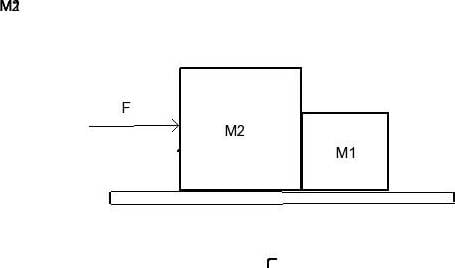# Force exerted by M1 on M2 problem

## Homework Statement

The illustration is not drawn to scale. M2 = 4 kg and M1=20kg. They're both on a frictionless surface. A 36N constant force is applied to M2. What's the force exerted by m1 on m2?F=ma

## The Attempt at a Solution

According to Newton's 3rd law, forces come in pairs. If there's 36N of force exerted on M2, the block should move and transfer the force onto M1. Since the surface is frictionless, I don't have to worry about any force being lost due to friction. Thus, 36N of force should be exerted onto M2 by M1. However, I know my logic is wrong, since the online quiz told me that the correct answer is 30N. How do I correctly approach this problem?

haruspex
Homework Helper
Gold Member
According to Newton's 3rd law, forces come in pairs.
Yes, but as action and reaction. You can use that to determine the relationship between the forces the two masses exert on each other.
Consider the acceleration of the system. What force therefore acts on m1?

Chestermiller
Mentor
Since M1 and M2 move together, if a force of 36 N is applied to them, what is their acceleration?

Chet

Hi JSmithDawg.:)
Consider m1 and m2 as a system and find the acceleration of the system by using F=ma ,as Chestermiller said. Then draw free body diagrams of m1 and m2 separately. Can you find the magnitude of force acting on m1 due to m2? What is the relation between the acting on m1 by m2 and force acting on m2 by m1?